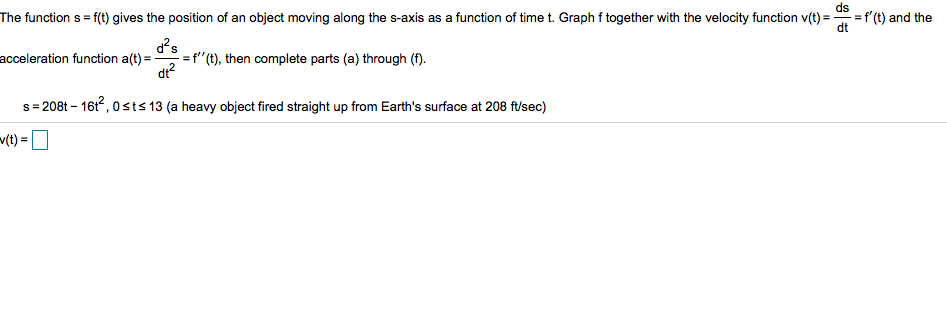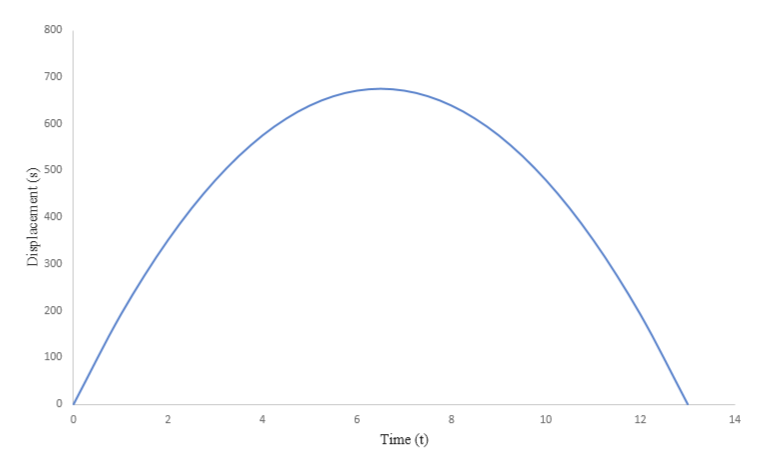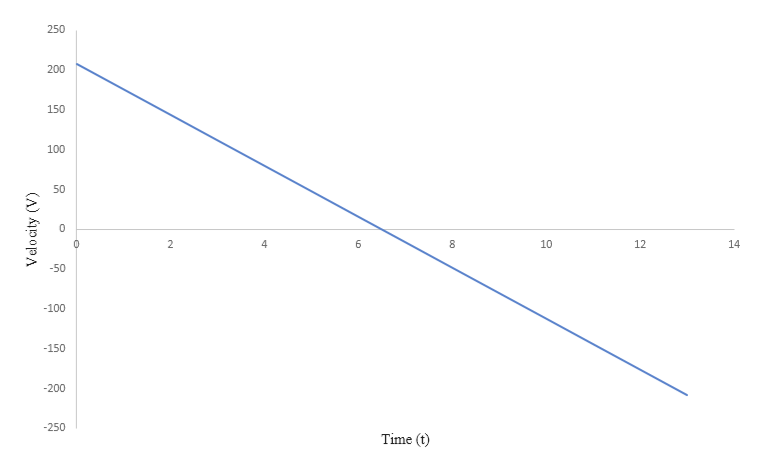# ds=f'(t) and theThe function s= f(t) gives the position of an object moving along the s-axis as a function of time t. Graph f together with the velocity function v(t)=d2sacceleration function al (t), then complete parts (a) through (f).s 208t 16t,0sts 13 (a heavy object fired straight up from Earth's surface at 208 ft/sec)(t)

Question
3 views

Can you help me step by step with this question pleasehelp_outlineImage Transcriptioncloseds =f'(t) and the The function s= f(t) gives the position of an object moving along the s-axis as a function of time t. Graph f together with the velocity function v(t)= d2s acceleration function al (t), then complete parts (a) through (f). s 208t 16t,0sts 13 (a heavy object fired straight up from Earth's surface at 208 ft/sec) (t) fullscreen
check_circle

Step 1

The position of an object with respect to time is S = 208t – 16t2, 0 ≤ t ≤ 13.
The graph of position vs time of given object is shown below:help_outlineImage Transcriptionclose800 700 600 500 400 300 200 100 0 8 0 6 10 12 Time (t Displacement (s) fullscreen
Step 2

The velocity of the object is given by differentiating the position with respect to time, it gives:

Step 3

The graph of velocity vs time of g...help_outlineImage Transcriptionclose250 200 150 100 50 2 4 6 8 10 12 14 -50 -100 -150 -200 -250 Time (t Velo city (V) fullscreen

### Want to see the full answer?

See Solution

#### Want to see this answer and more?

Solutions are written by subject experts who are available 24/7. Questions are typically answered within 1 hour.*

See Solution
*Response times may vary by subject and question.
Tagged in